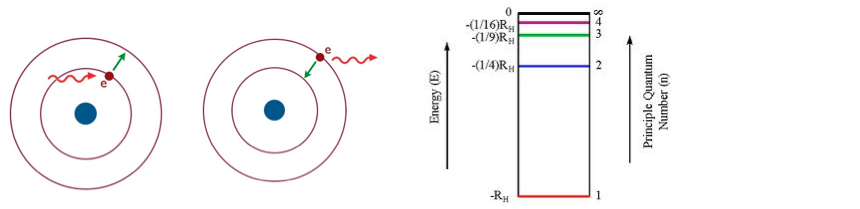# Problem: Which of the following transitions (in a hydrogen atom) represent  emission of the smallest or shortest wavelength? n = 4 to n = 2n = 3 to n=  4n = 1 to n = 2n = 7 to n = 5n = 2 to n = 5

###### FREE Expert Solution
92% (433 ratings)
###### Problem Details

Which of the following transitions (in a hydrogen atom) represent  emission of the smallest or shortest wavelength?

1. n = 4 to n = 2
2. n = 3 to n=  4
3. n = 1 to n = 2
4. n = 7 to n = 5
5. n = 2 to n = 5What scientific concept do you need to know in order to solve this problem?

Our tutors have indicated that to solve this problem you will need to apply the Bohr Model concept. You can view video lessons to learn Bohr Model. Or if you need more Bohr Model practice, you can also practice Bohr Model practice problems.

What is the difficulty of this problem?

Our tutors rated the difficulty ofWhich of the following transitions (in a hydrogen atom) repr...as medium difficulty.

How long does this problem take to solve?

Our expert Chemistry tutor, Jules took 4 minutes and 12 seconds to solve this problem. You can follow their steps in the video explanation above.

What professor is this problem relevant for?

Based on our data, we think this problem is relevant for Professor Gunasekera's class at UIUC.